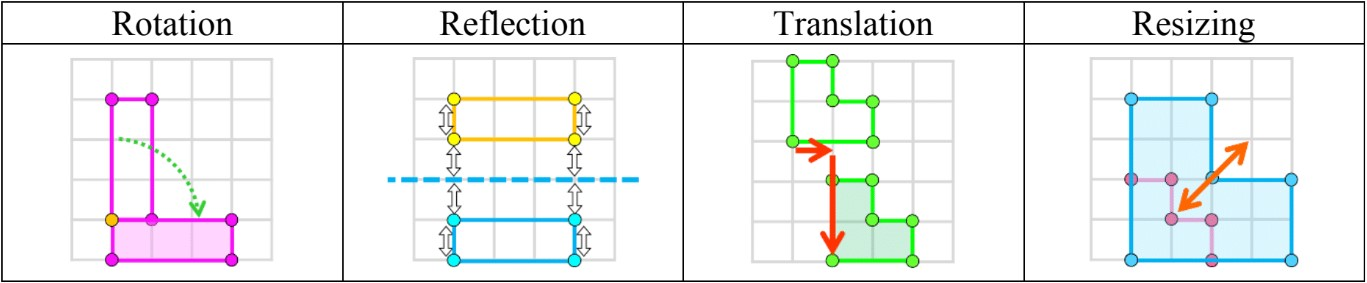# Basics of coordinate plane#### All in One Place

Everything you need for better grades in university, high school and elementary.#### Learn with Ease

Made in Canada with help for all provincial curriculums, so you can study in confidence.#### Instant and Unlimited Help

0/17
##### Examples
###### Lessons
1. Drawing a point on the coordinate plane
Draw a point for the coordinates given:
1. (2,3)
2. (8,6)
3. (4,9)
4. (7,5)
2. Naming the coordinates of a point
Write the coordinates for the point in the format ($x$,$y$)
1.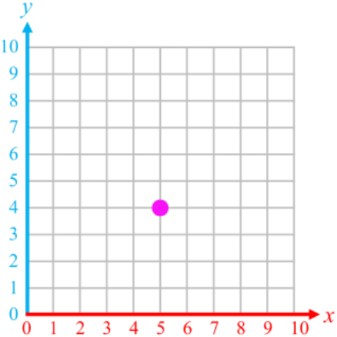2.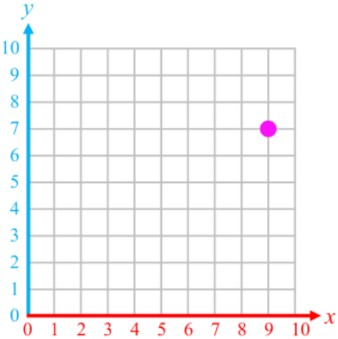3.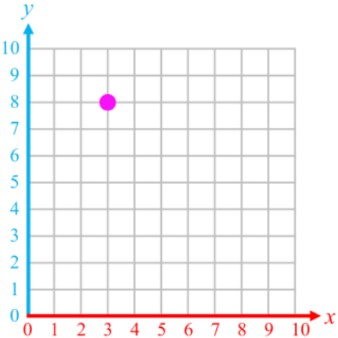4.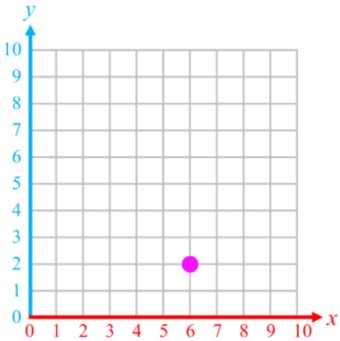3. Translations of points on the coordinate plane
Slide the point following the instructions. Draw the new point and write its coordinates.
1. move 8 units left and 3 units up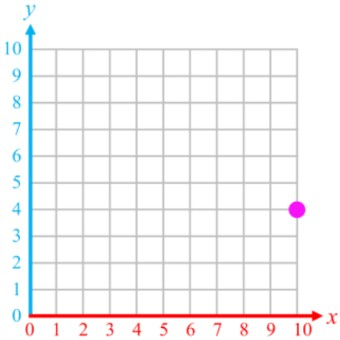2. move 0 units left and 6 units down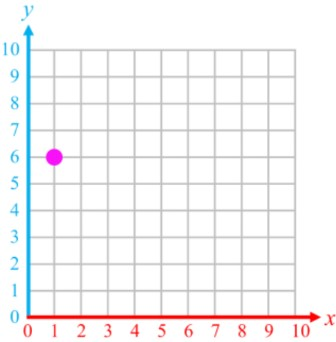3. move 3 units right and 1 unit up4. Polygons and coordinate grids
Draw and name the missing point to complete the shape
1. rectangle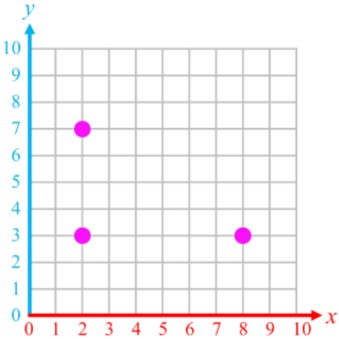2. hexagon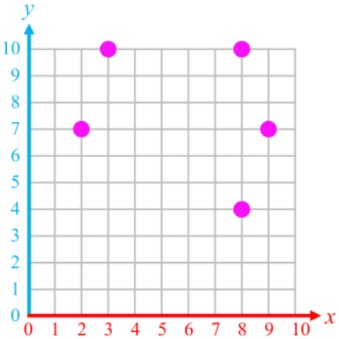3. octagon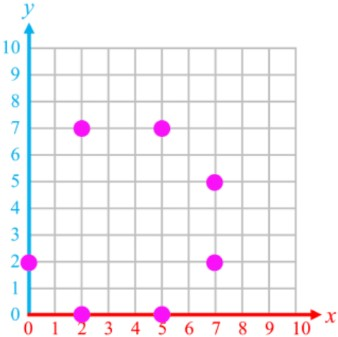5. Basic transformations on coordinate grids
The rectangle has points (2,7), (4,7), (2,3), (4,3)
1. Translate the whole shape by sliding 5 units right and 2 units down. Draw all the points and sides after the translation. Give the coordinates of the new vertex points.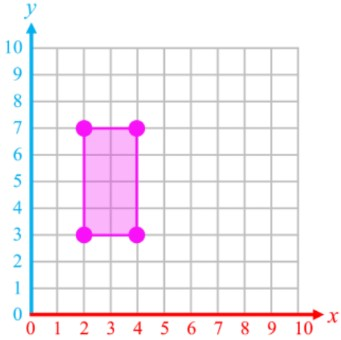2. Rotate the polygon 90° clockwise around the point (4,3). Draw all the points and sides after the rotation. Give the coordinates of the new vertex points.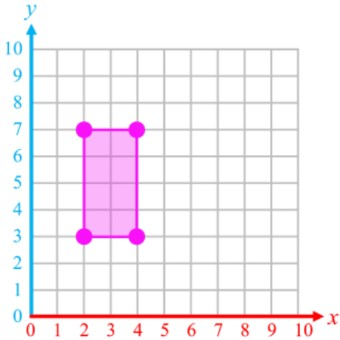3. Reflect the shape across the dotted line. Draw all the points and sides after the reflection. Give the coordinates of the new vertex points.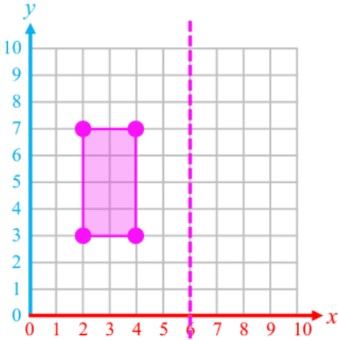###### Topic Notes

In this lesson, we will learn:

• How to use the coordinate plane system to draw and locate points in the format ($x$, $y$)
• How to rotate, reflect, and translate shapes on the coordinate plane

Notes:

• The coordinate plane (also called coordinate system or the 2D cartesian plane/system) is a system where points on a grid are given locations called "coordinates"

• Example: use a simple dot grid (or array) to understand how the coordinate plane works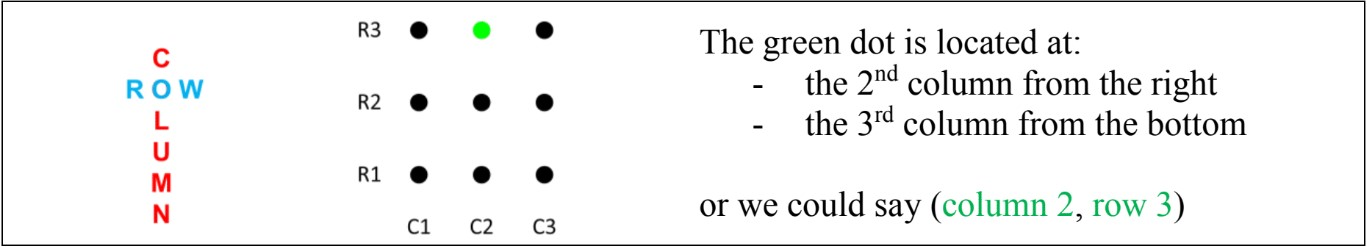• The unique and specific location of any point is given by two pieces of information
• the column number (from left to right)
• and the row number (from top to bottom)

• The coordinate plane has two dimensions and points are given in the format point (x, y)
• the location on the x-axis (from left to right, the column number)
• and the location of the y-axis (from top to bottom, the row number)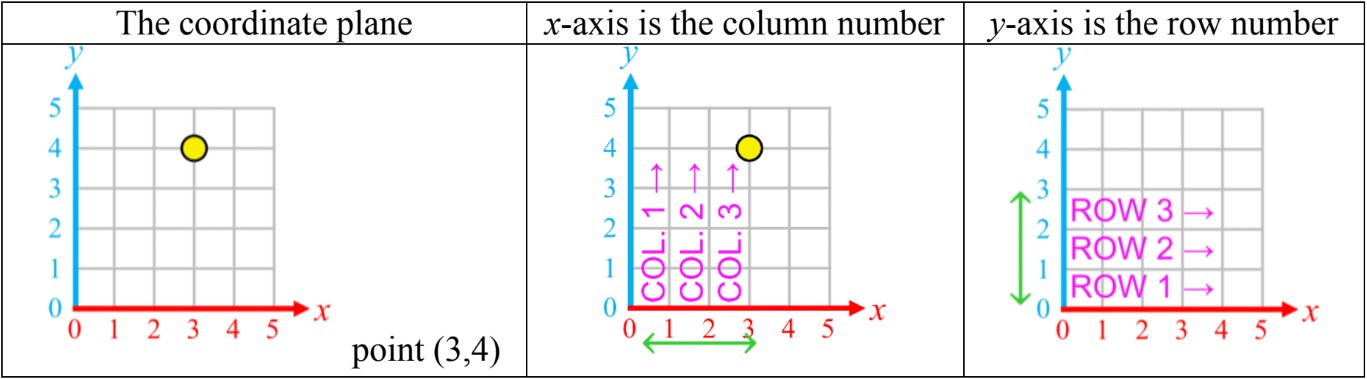• There are four types of transformations that can be done on the coordinate plane
• Rotations: spinning shapes around a point
• Reflections: creating a mirror image across a mirror line
• Translations: sliding a shape (left $\longleftrightarrow$ right) and/or (up $\updownarrow$ down)
• Resizing: stretching or shrinking a shape by a scale factor (i.e. doubling, halving)